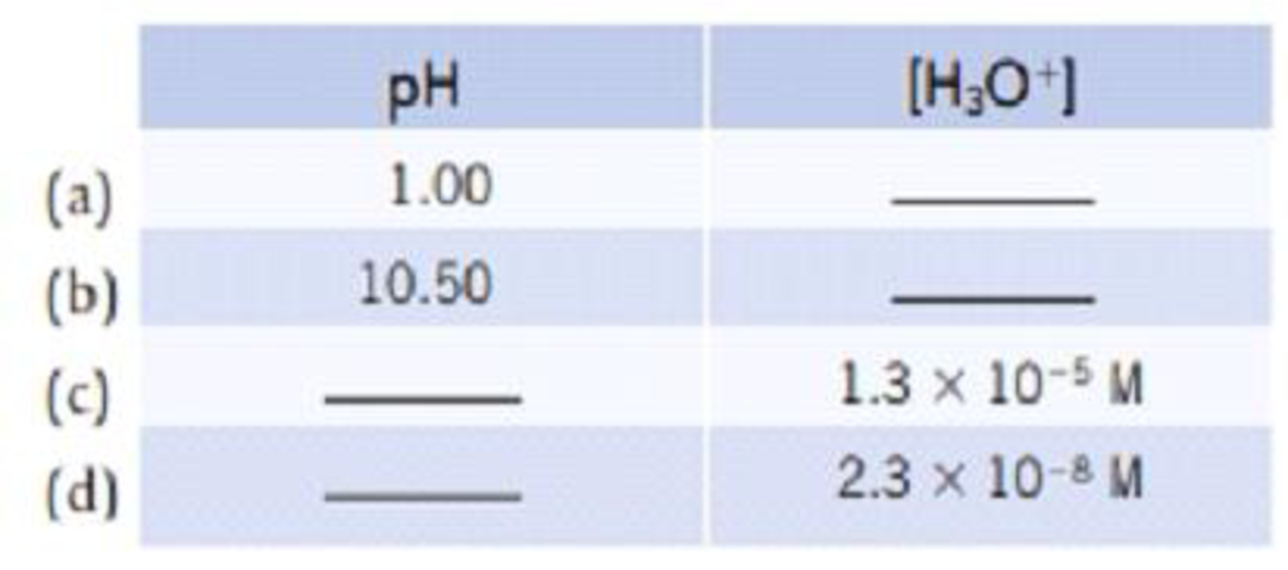Chapter 4, Problem 59PS

Chapter
Section
Textbook Problem

Make the following conversions. In each case, tell whether the solution is acidic or basic.(a)

Interpretation Introduction

Interpretation:

The pH of 1.00 has to be converted into [H3O+] and whether it is acidic or basic has to be determined.

Concept introduction:

• pH of a solution is the negative of the base -10 logarithm of the hydronium ion concentration.

pH=-log[H3O+]

• Concentration of hydronium ion [H3O+]=10-pH
• For an acidic solution pH<7 and for a basic solution pH>7.
Explanation

The given solution has a pH of 1.00 and its hydronium ion concentration can be determined by using the equation,

[H3O+]=10-pH

Substituting pH value of solution a in the above equation,

[H3O+]=10-(1

(b)

Interpretation Introduction

Interpretation:

The pH of 10.50 has to be converted into [H3O+] and whether it is acidic or basic has to be determined.

Concept introduction:

• pH of a solution is the negative of the base -10 logarithm of the hydronium ion concentration.

pH=-log[H3O+]

• Concentration of hydronium ion [H3O+]=10-pH
• For an acidic solution pH<7 and for a basic solution pH>7.

(c)

Interpretation Introduction

Interpretation:

The [H3O+] has to be converted into pH and whether it is acidic or basic has to be determined.

Concept introduction:

• pH of a solution is the negative of the base -10 logarithm of the hydronium ion concentration.

pH=-log[H3O+]

• Concentration of hydronium ion [H3O+]=10-pH
• For an acidic solution pH<7 and for a basic solution pH>7.

(d)

Interpretation Introduction

Interpretation:

The [H3O+] has to be converted into pH and whether it is acidic or basic has to be determined.

Concept introduction:

• pH of a solution is the negative of the base -10 logarithm of the hydronium ion concentration.

pH=-log[H3O+]

• Concentration of hydronium ion [H3O+]=10-pH
• For an acidic solution pH<7 and for a basic solution pH>7.

Still sussing out bartleby?

Check out a sample textbook solution.

See a sample solution

The Solution to Your Study Problems

Bartleby provides explanations to thousands of textbook problems written by our experts, many with advanced degrees!

Get Started